# Types of Variables in a Class in Python

## Types of Class Variables in Python with Examples

In this article, I am going to discuss Types of Class Variables in Python with examples. Please read our previous article where we discussed Constructors in Python with examples. As part of this article, we are going to discuss the following pointers which are related to Class Variables in Python.

1. Types of Class Variables in Python
2. Instance Variables in Python
3. Where instance variables can be declared?
4. Accessing instance variables in Python
5. Static Variables in Python
6. Declaring static variables in Python
7. Accessing a static variable in Python
8. Local Variables in Python
##### Types of Class Variables in Python:

Inside a class, we can have three types of variables. They are:

1. Instance variables (object level variables)
2. Static variables (class level variables)
3. Local variables
##### Instance Variables in Python:

If the value of a variable is changing from object to object then such variables are called as instance variables.

Example: (Instance Variables) demo10.py

```class Student:
def __init__(self, name, id):
self.name=name
self.id=id

s1=Student('Nitya', 1)
s2=Student('Anushka', 2)
print("Studen1 info:")
print("Name: ", s1.name)
print("Id : ", s1.id)
print("Studen2 info:")
print("Name: ",s2.name)
print("Id : ",s2.id)```

Output: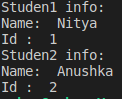The value of id,name is different for instances(objects) s1 and s2, hence they are instance variables. In the case of instance variables for every object a separate copy will be created.

##### Where instance variables can be declared?

We can declare and initialize the instance variables in following ways,

1. By using a constructor.
2. By using instance methods.
3. By using object name
##### Declaring instance variables in a constructor

We can declare and initialize instance variables inside a constructor by using self variable.

Example: Declaring instance variables in a constructor (demo11.py)

```class Employee:
def __init__(self):
self.eno=1
self.ename="Nithya"
self.esal=100
e=Employee()

print("Employee number: ",e.eno)
print("Employee name: ", e.ename)
print("Employee salary: ", e.esal)
print(e.__dict__)```

Output: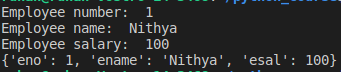Every object in Python has an attribute denoted as __dict__, which python creates internally. This displays the object information in dictionary form. It maps the attribute name to its value.

##### Declaring instance variables using instance methods

We can declare and initialize instance variables inside instance method by using self variable.

Example: Declaring instance variables using instance methods (demo12.py)

```class Student:
def m1(self):
self.a=11
self.b=21
self.c=34
print(self.a)
print(self.b)
print(self.c)

s= Student()
s.m1()
print(s.__dict__)```

Output: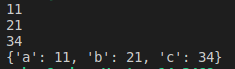##### Declaring instance variables using object name

We can declare and initialize instance variables by using object name as well

Example: Declaring instance variables using object name (demo13.py)

```class Test:
def __init__(self):
print("This is constructor")
def m1(self):
print("This is instance method")

t=Test()
t.m1()
t.a=10
t.b=20
t.c=55
print(t.a)
print(t.b)
print(t.c)
print(t.__dict__)```

Output: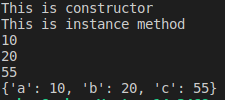##### Accessing instance variables in Python:

The instance variable can be accessed in two ways:

1. By using self variable
2. By using object name
##### Accessing instance variables By self variable in Python:

We can access instance variables within the class by using self variable.

Example: Accessing instance variables in Python By self variable (demo14.py)

```class Student:
def __init__(self):
self.a=10
self.b=20
def display(self):
print(self.a)
print(self.b)
s= Student()
s.display()```

Output: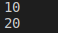##### Accessing instance variables By object name:

We can access instance variables by using the object’s name.

Example: Accessing instance variables in Python By object name (demo15.py)

```lass Student:
def __init__(self):
self.a=10
self.b=20

t=Student()
print(t.a)
print(t.b)```

Output: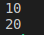##### Static Variables in Python

If the value of a variable is not changing from object to object, such types of variables are called static variables or class level variables. We can access static variables either by class name or by object name. Accessing static variables with class names is highly recommended than object names.

##### Accessing static variables outside of class

Example: Accessing static variables outside of class in Python (demo16.py)

```class Student:
college_name='GITAM'
def __init__(self, name, id):
self.name=name
self.id=id
s1=Student('Nithya', 1)
s2=Student('Anushka', 2)

print("Studen1 info:")
print("Name: ", s1.name)
print("Id : ", s1.id)
print("College name n : ", Student.college_name)

print("\n")
print("Studen2 info:")
print("Name: ",s2.name)
print("Id : ",s2.id)
print("College name : ", Student.college_name)```

Output: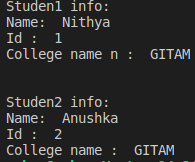Example: Accessing static variables with object name (demo17.py)

```class Student:
college_name='GITAM'
def __init__(self, name, id):
self.name=name
self.id=id
s1=Student('Nithya', 1)
s2=Student('Anushka', 2)

print("Studen1 info:")
print("Name: ", s1.name)
print("Id : ", s1.id)
print("College name n : ", s1.college_name)

print("\n")
print("Studen2 info:")
print("Name: ",s2.name)
print("Id : ",s2.id)
print("College name : ", s1.college_name)```

Output:##### Declaring static variables in Python:

We can declare static variable in the following ways,

1. Inside class and outside of the method
2. Inside constructor
3. Inside instance method
4. Inside class method
5. Inside static method
##### Declaring static variable inside class and outside of the method

Generally, we can declare and initialize static variable within the class and outside of the methods. This is the preferred way.

Example: Declaring static variable inside class and outside of the method (demo18.py)

```class Demo:
a=20
def m(self):
print("this is method")
print(Demo.__dict__)```

Output: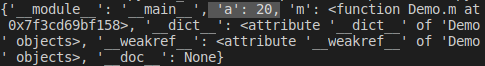##### Declaring static variable inside constructor

We can declare and initialize static variables within the constructor by using class’s name.

Example: Declaring static variable inside constructor (demo19.py)

```class Demo:

def __init__(self):
Demo.b=20
d = Demo()
print(Demo.__dict__)```

Output: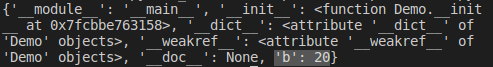##### Declaring static variable inside instance method

We can declare and initialize static variable inside instance method by using class Name just as we have done in the constructor.

Example: Declaring static variable inside instance method (demo20.py)

```class Demo:

def m1(self):
Demo.b=20
obj=Demo()
obj.m1()
print(Demo.__dict__)```

Output: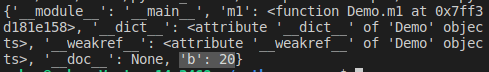##### Declaring static variable inside class method

We can declare and initialise static variable inside class method in two ways, one is using class name, other is using cls pre-defined variable

##### What is class method?

A method inside a class with @classmethod decorator is called a class method. We will discuss this in next chapters. Inside the class method we can declare and initialize static variables by using the class’s name.

Example: Declaring static variable inside class method (demo21.py)

```class Demo:
@classmethod
def m2(cls):
Demo.b=30
obj=Demo()
obj.m2()
print(Demo.__dict__)```

Output: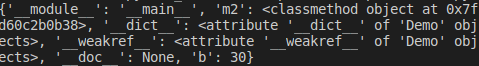We can also do the initialization in the class method using the cls variable. cls is predefined variable in python. We should pass the cls parameter to the class methods just as we use self for instance methods.

Example: static variable inside class method by using cls (demo21.py)

```class Demo:
@classmethod
def m2(cls):
cls.b=30
obj=Demo()
obj.m2()
print(Demo.__dict__)```

Output: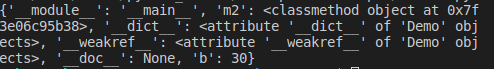##### Declaring static variable inside static method

We can declare and initialize static variables inside a static method by using class name.

##### What is a static method?

Before method if we write @staticmethod decorator then that method will become a staticmethod. We will learn more about static method in upcoming chapter

Example: static variable inside static method by using class name (demo22.py)

```class Demo:
@staticmethod
def m3():
Demo.z=10
Demo.m3()
print(Demo.__dict__)```

Output: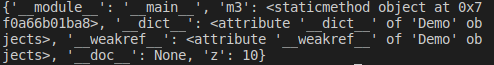##### Accessing a static variable within the class in Python:

We have already discussed with examples, how static variables can be accessed outside of class earlier. Now, we shall discuss how we can access static variable in (within the class),

1. Inside constructor
2. Inside instance method
3. Inside class method
4. Inside static method
##### Accessing a static variable Inside Constructor:

We can access static variables inside the constructor by using either self or class name.

Example: Accessing static variable Inside Constructor (demo23.py)

```class Demo:
a=10
def __init__(self):
print(self.a)
print(Demo.a)

d = Demo()```

Output: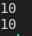##### Accessing a static variable Inside instance method:

We can access static variables inside instance methods by using either self or class name.

Example: Accessing static variable Inside instance method (demo24.py)

```class Demo:
a=10
def m1(self):
print(self.a)
print(Demo.a)
obj=Demo()
obj.m1()```

Output:##### Accessing a static variables Inside class method:

We can access static variables inside class methods by using cls variables and class’s name.

Example: Accessing static variable Inside class method (demo25.py)

```class Demo:
a=10
@classmethod
def m1(cls):
print(cls.a)
print(Demo.a)
obj=Demo()
obj.m1()```

Output: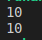##### Accessing a static variables Inside static method:

We can access static variables inside the static method by using class’s name.

Example: Accessing static variable Inside static method (demo26.py)

```class Demo:
a=10
@staticmethod
def m1():
print(Demo.a)
obj=Demo()
obj.m1()```

Output: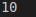##### Local Variables in Python:

The variable which we declare inside of the method is called a local variable. Generally, for temporary usage we create local variables to use within the methods. The scope of these variables is limited to the method in which they are declared. They are not accessible out side of the methods.

Example: Local Variables in Python (demo27.py)

```class Demo:
def m(self):
a=10 #Local Variable
print(a)
d=Demo()
d.m()```

Output:Example: Local Variables in Python (demo28.py)

```class Demo:
def m(self):
a=10 #Local Variable
print(a)

def n(self):
print(a) #'a' is local variable of m()
d=Demo()```

Output: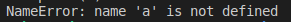### 1 thought on “Types of Variables in a Class in Python”

1.class Demo:
def m(self):
a=10 #Local Variable
print(a)
def n(self):
print(a) #’a’ is local variable of m()
d=Demo()

In this the following line is missed at the end of the program (demo28):
d.n()